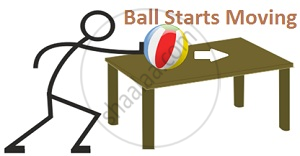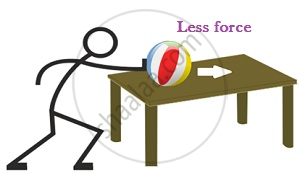Share

# Newton'S First Law of Motion

## Newton’s First law of motion

A body at rest tends to remain at rest and a body in uniform motion tends to remain in the state of uniform motion until & unless an external force is applied on it.

For example, a ball lying on the table at rest will remain at rest until an external force is applied on it.Balanced & Unbalanced forces :-Balanced Forces:

• Equal & Opposite forces

• Do not cause any change in motion

Unbalanced Forces:

• Unequal forces

• Can be in the same or opposite direction

• Causes a change in motionFor example, in Tug of war, If teams 1 & 2 apply equal forces in opposite directions, there would be no net force. This is Balanced force.
However, if Team 1 exerts more force than Team 2, then there would be a net movement towards Team 1 and Team 1 would win. This is unbalanced force.

Momentum
Newton’s first law was for scenarios where net force = 0. The second law is for scenarios with net force not equal to 0. Momentum plays a crucial role in Second law.

• Momentum is the product of mass of a body & its velocity

• It is a Vector quantity

• It is denoted by p = mv

For example, A ball of 1 kg moving with 10m/sec has a momentum 10kg m/sec.

Momentum of a system remains conserved. Therefore,

• Greater force is required to set heavier bodies in motion• Greater force is required to stop bodies moving with higher velocitiesConclusion:
Greater the change in momentum in a given time, greater is the force that needs to be applied. In other words, greater the change in momentum vector, greater is the force applied.

S